## GAT Quantitative Part-1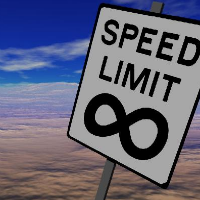GAT Quantitative Part-1 GAT Quantitative Part-1 Word Problems (Speed, Distance & Time) In past, have you ever read physics? If yes, you must know a formula that shows relationship between ‘Distance’, ‘Speed’ and ‘Time’ as shown bellow: Distance = Speed × Time Also,       and       Read More …

## Basic Math Part-7Basic Math Part-7 Basic Math Part-7 Practice Exercise (Drills) These drill sets are made with intention to provide the topic-wise practice exercise to make the important points into your figure tips and avoid silly mistakes. If you get full grip on your calculation skill, you can get perform much better Read More …

## Basic Math Part-6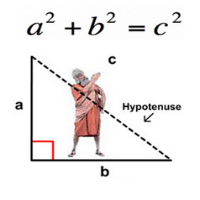Basic Math Part-6 Basic Math Part-6 Geometry Lines & Angles: When we joint the two different points straight-way it makes a line. A line can be of different directions i.e., horizontal lines and vertical lines. Horizontal lines are flat in direction, like surface of water; while vertical lines are steep Read More …

## Basic Math Part-5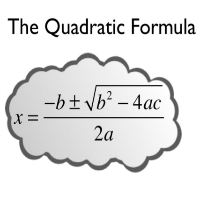Basic Math Part-5 Basic Math Part-5 Algebra (Solving Equations) Equations: Any expression that includes an equality sign ‘=‘ and either a variable x, ‘x2, y etc’ or a constant value ‘2, 3 or 4 etc.’ is known as equation. x + y = 5, 2x + y = 15 and Read More …

## Basic Math Part-4Basic Math Part-3 Basic Math Part-4 Arithmetic (Factors, Multiples & Percents) Factors & Multiples: Before proceeding further, it’s very important to know the difference and relationship between factors and multiples. Many students confuse at the start of the course to distinguish between these two. First, we’re discussing these separately, after Read More …

## Basic Math Part-3Basic Math Part-3 Basic Math Part-3 Arithmetic (PEDMAS) Fractions & Decimals: We already have discussed what are fractions and decimals. Here we’re going to describe the application of these terms in math. Now, we need to learn the parts of Fractions as well as decimals.   Parts of Fractions: There Read More …

## Basic Math Part-2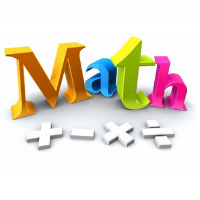Basic Math Part-2 Basic Math Part-2 Arithmetic (Arithmetic Operations ‘+, –, ×, ÷’) Multiplication: We have learned how to add numbers. If we add same number many times, it’s called multiplication. For instance, If we add 3, by 5 times, i.e., 3 + 3 + 3 + 3 + 3 Read More …

## Basic Math Part-1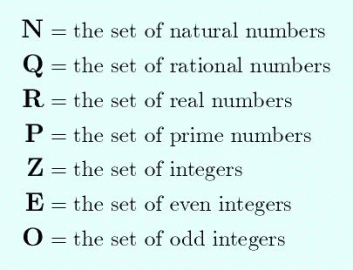Basic Math Part-1 Basic Math Part-1 Arithmetic (Digits & Numbers) Digits: There are total ten digits: 0, 1, 2, 3, 4, 5, 6, 7, 8 and 9. These are the basic elements of math that combines to form many numbers. The whole math revolves around these ten digits.   Numbers: Read More …

## GAT FREE Quantitative Practice Question 1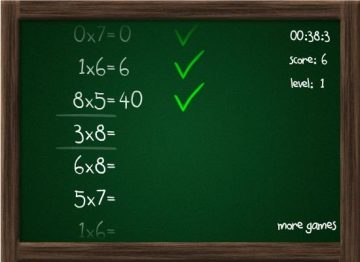GAT FREE Quantitative Practice Question 1 GAT FREE Quantitative Practice Question 1 Firstly, Today’s GAT FREE Quantitative Practice Question 1 is a problem solving question on percentages. Try solving it first before you look at the explanation below. Question: Islamabad is the capital city of Pakistan. If the population of Read More …